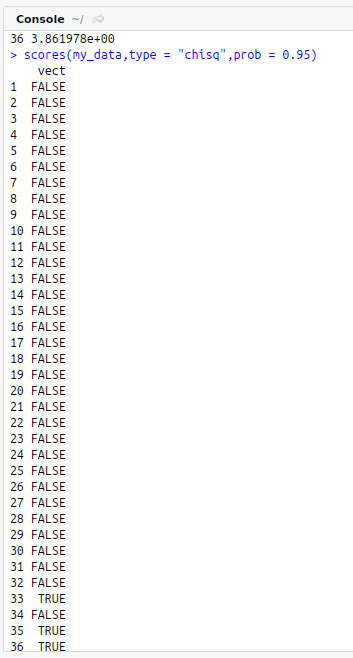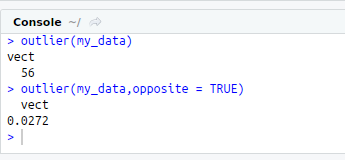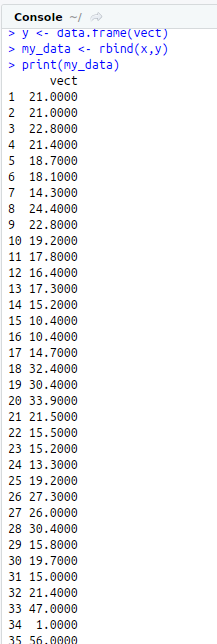• #5, First Floor, 4th Street Dr. Subbarayan Nagar Kodambakkam, Chennai-600 024 Landmark : Samiyar Madam
• pro@slogix.in
• +91- 81240 01111

#### Social List

##### How to resolve Outliers using R?
###### Description

To resolve the outliers in R programming.

###### Process
What is Outlier?

Outliers are points that are distant from remaining observations.

Need of detecting Outliers:

They can potentially skew or bias any analysis performed on the dataset.

It is therefore very important to detect and adequately deal with outliers.

Package and Function:

R Package : outliers

R Function : outlier(x,opposite =) --

Extreme most observation from the mean.

X -- Data Frame

Opposite -- logical value, fetches outlier from the other side.

R Function : scores(x,type= chi-sq,z,t )

Type -- Normalized Scores based on type.

###### Sapmle Code

#Resolving Outlier

#Detecting Outlier

#install.packages(“outliers”)

library(“outliers”)

#Input

vect<-mtcars\$mpg

x<-data.frame(vect)

print(x)

#Add some dummy outlier to input data

vect<-c(47,1,56,0.0272)

y<-data.frame(vect)

my_data<-rbind(x,y)

print(my_data)

#Outlier

outlier(my_data)

#Outlier from other side

outlier(my_data,opposite = TRUE)

#Visualizing Outlier using Boxplot

boxplot(my_data,notch=TRUE,col=”green”,main=”BoxPlot with Outlier”)

#Chi-Squared Scores

scores(my_data,type = “chisq”)

#Beyond 95th Percentile based on Chi-Square

scores(my_data,type = “chisq”,prob = 0.95)

#Replacing Outlier with NA values

remove_outliers<-function(x,na.rm=TRUE) {

qnt<-quantile(x, probs=c(.25, .75))

H<-1.5 * IQR(x, na.rm = na.rm)

y<-x

y[x < (qnt – H)]<-NA

y[x > (qnt + H)]<-NA

y

}

r<-remove_outliers(my_data\$vect)

print(as.data.frame(r))

print(my_data)

#Box Plot witout Outlier

boxplot(r,notch=TRUE,col=”green”,main=”Box Plot without Outliers”)

###### Screenshots